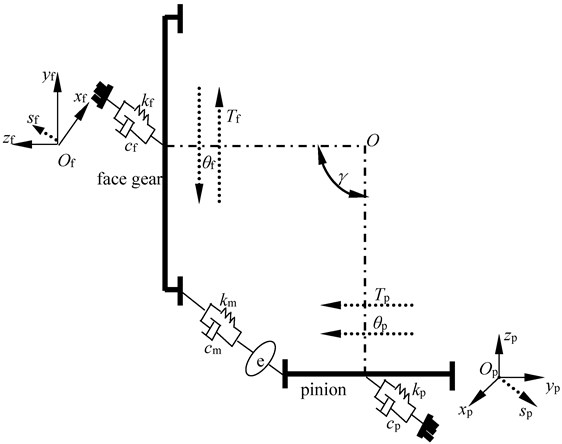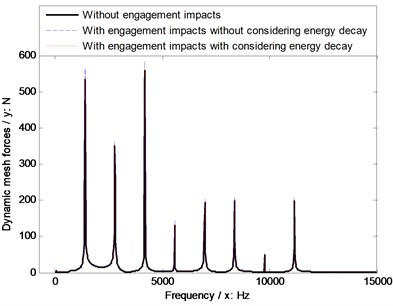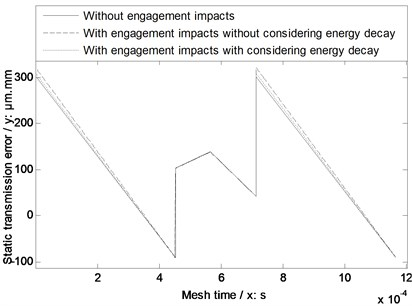Published: 08 December 2016

# Constructed solutions of face gear dynamics associated with engagement impact energy decay

Zhengminqing Li1
Hao Wu2
Rupeng Zhu3
1, 2, 3Nanjing University of Aeronautics and Astronautics, Nanjing, China
Corresponding Author:
Zhengminqing Li
Views 30

#### Abstract

Face gear drives are focused by many researchers, due to several strong-points versus traditional spiral bevel gear drives. However, calculation solutions of face gear dynamics associated with engagement impact energy decay are not to be constructed, according to the limited published issues. Thus, in the study, a calculation solution of engagement impact energy decay of face gear drives is proposed, a face gear dynamic model is established, and the dynamic mesh forces of an example case of face gear drives without engagement impacts as well as with engagement impacts and engagement impact suppressions are simulated. The results indicate the fidelity of the proposed calculation solutions of face gear dynamics associated with engagement impact energy decay could be accepted. These contributions would improve the developments of face gear drive modifications and engineering applications of face gear drives in the future.

## 1. Introduction

Face gear drives are addressed by scholars, due to several strengths versus spiral bevel gear drives [1-15], and according to research achievements of Litvin et. al. [16-21], face gear drives are suggested to be used in first-stage gear drives of helicopter main gear boxes. Due to operating characteristics of first-stage gear drives, namely, input-stage gear drives in helicopter main gear boxes, face gear dynamics is focused by many researchers [22-27]. However, according to the limited published issues about face gear dynamics, calculation solutions of face gear dynamics associated with engagement impact energy decay are not to be constructed. Thus, in the study, an engagement impact energy decay calculation solution of face gear drives is proposed, and a four degree-of-freedom (DOF) face gear dynamic model is formulated. Furthermore, the dynamic mesh forces of an example case of face gear drives without considering engagement impacts, as well as with considering engagement impacts and engagement impact suppressions are simulated. The results indicate the fidelity of the proposed calculation solutions of face gear dynamics associated with engagement impact energy decay could be accepted. These contributions would benefit to construct face gear drive modification solutions, and improve engineering applications of face gear drives in the future.

## 2. Solutions of face gear dynamics associated with engagement impact energy decay

### 2.1. Calculation solution of engagement impact energy with considering energy decay

According to the reference , Li and Zhu proposed the calculation solutions of the engagement impact energy and the conversion between engagement impact energy and static transmission errors (STE) of face gear drives. But, they neglect energy decay of engagement impact contact interfaces.

Thus, according to the reference , engagement impact energy of face gear drives with considering energy decay can be derived by:

1
${E}_{d}=1+\sqrt{1+\left[{\left(E-1\right)}^{2}-1\right]\left(1+\frac{{m}_{e}}{{m}_{p}}\right)},$

where $E$ is an engagement impact energy, which can be calculated according to the reference , ${E}_{d}$ is an engagement impact energy with considering energy decay, ${m}_{e}$ is a quality of face gear drives, and ${m}_{p}$ is a quality of pinions.

### 2.2. Four DOF dynamic model

A four DOF dynamic model of face gear drives, as shown in Fig. 1, is formulated for assessing the fidelity of the proposed calculation solutions of face gear dynamics associated with engagement impact energy decay.

Fig. 1A four-DOF dynamic modelAs illustrated in Fig. 1, mathematic equations of the dynamic model can be deduced as:

2
$\left\{\begin{array}{l}{m}_{p}{s}_{p}^{\text{'}\text{'}}+{c}_{p}{s}_{p}^{\text{'}}+{k}_{p}{s}_{p}=-{F}_{m},\\ {m}_{f}{s}_{f}^{\text{'}\text{'}}+{c}_{f}{s}_{f}^{\text{'}}+{k}_{f}{s}_{f}={F}_{m},\\ {I}_{p}{\theta }_{p}^{\text{'}\text{'}}+{F}_{m}{R}_{bp}={T}_{p},\\ {I}_{f}{\theta }_{f}^{\text{'}\text{'}}+{F}_{m}{R}_{bf}=-{T}_{f},\end{array}\right\$

where ${F}_{m}$ can be written in:

3
${F}_{m}={k}_{m}\mathrm{s}\mathrm{i}\mathrm{n}\left(\gamma \right)\left({s}_{p}-{s}_{f}+{R}_{bp}{\theta }_{p}-{R}_{bf}{\theta }_{f}-e\right)$
$+{c}_{m}\mathrm{s}\mathrm{i}\mathrm{n}\left(\gamma \right)\left({s}_{p}^{\text{'}}-{s}_{f}^{\text{'}}+{R}_{bp}{\theta }_{p}^{\text{'}}-{R}_{bf}{\theta }_{f}^{\text{'}}-{e}^{\text{'}}\right),$

where $\theta$ is a torsion degree of freedom, $s$ is a bending degree of freedom, $T$ is a torsion, $k$ is a bending stiffness, $c$ is a bending damping, $m$ is a quality, $I$ is a moment of inertia, ($\text{'}$) is first derivative, ($\text{'}\text{'}$) is second derivative, subscript $f$ and $p$ express a face gear and a pinion respectively. In addition, ${k}_{m}$ is mesh stiffness, ${c}_{m}$ is mesh damping, and $e$ is a comprehensive meshing error.

## 3. Simulations

In order to investigate dynamic mesh force differences of face gear drives among three conditions, namely, without engagement impacts, with engagement impacts, and with engagement impacts associated with impact energy decay, as well as evaluate the fidelity of the proposed calculation solutions of face gear dynamics associated with engagement impact energy decay, geometric parameters, operating conditions and material characteristics of an example case of face gear drives are given in Table 1.

Table 1Parameters of an example case

 Names Values Units Geometric parameters Modulus 2.5 mm Pressure angle 22.5 ° Tooth number of pinions 21 – Drive ratio 3.476 – Shaft angle 87 ° Tooth number difference between pinions and generation gears 1 – Addendum coefficient 1 – Clearance coefficient 0.25 – Operating conditions Power 50 kW Input rotation speed 4000 r/min Material characteristics Modulus of elasticity 210000 MPa Poisson ratio 0.3 –

Based on the parameters, listed in Table 1, the STE, which can be calculated according to the reference , of the example case among three conditions, meaning, without engagement impacts, with engagement impacts, and with engagement impacts associated with impact energy decay, are simulated, as shown in Fig. 2.

Fig. 3A dynamic mesh force simulation of the example caseFig. 2A simulation of STE of the example caseIntroducing the results of Fig. 2 into Eq. (2), the dynamic mesh forces of the example case of face gear drives among three conditions are simulated, as shown in Fig. 3.

As illustrated in Fig. 2 and Fig. 3, the STE and dynamic mesh force simulation results with considering engagement impacts associated with energy decay are between the simulation results without engagement impacts and those with engagement impacts, in which energy decay is not considered, which is consistent with the general knowledge and phenomenon of face gear dynamics. Thus, the fidelity of the proposed calculation solutions of face gear dynamics associated with engagement impact energy decay could be accepted.

## 4. Conclusions

In this study, the most important work is to construct calculation solutions of face gear dynamics associated with engagement impact energy decay. Secondly, the fidelity of the proposed calculation solutions could be accepted according to the simulation results of an example case of face gear drives, which is fit for general cognitions of gear dynamics. These contributions would benefit to improve face gear drive modifications and engineering applications of face gear drives in the future.

04 April 2016
Accepted
20 May 2016
Published
08 December 2016
SUBJECTS
Mechanical vibrations and applications
Keywords
face gear drives
gear dynamics
dynamic mesh forces
engagement impact energy decay
static transmission errors
Acknowledgements

The authors are grateful for the financial support provided by the National Natural Science Foundation of China under No. 51105194 and No. 51375226, and the Fundamental Research Funds for the Central Universities under No. NS2015049. In addition, the authors declare that there is no conflict of interests regarding the publication of this article.